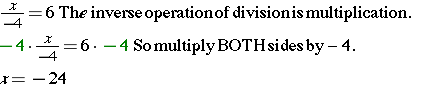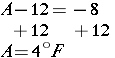# Solve Equations with IntegersIn this lesson, you will learn to combine the rules for solving integer operations problems with the one rule for solving a one-step equation. Remember, to solve a one-step equation, you will only need to perform one step and that will be the inverse operation.

Inverse Operations are operations that “undo” each other.

You know that a mathematical sentence that has an equal sign is known as an equation. 2 * 6 = 12 is an example of an equation.

Well, add at least one variable to that sentence and you have an algebraic equation.

2x = 4 is an example of an algebraic equation.

To solve an algebraic equation, it is necessary to isolate the variable by using inverse operations and properties of equality.

Look at this example of the inverse property of addition!

The sum of a number and its opposite is 0 5 + (-5) = 0 x + (-x) = 0

### Example 1Solve an addition equation with negative and positive numbers.-4 + 14 = 10 (Substitute your solution into the original equation and evaluate.)

.

### Example 2Solve a subtraction equation.Substitute your solution into the original equation and evaluate.

### Example 3Solve a multiplication equation.Substitute your solution into the original equation and evaluate.
2(-3) = -6 … that is correct!

### Example 4Solve a division equation.

Substitute your solution into the original equation and evaluate.

In many cases, it is necessary to write algebraic equations in order to solve word problems.

## Investigate

Bennett has 15 coins in his coin collection. That is 5 more than his friend Matthew has in his coin collection. Write an equation that represents m, the number of coins Matthew has.

Strategy

Now is the time to translate the words to math, using key words.

The words “more than” indicate addition.

The word “is” indicates where to place the equal sign.

• 15 coins         is         5 more than Matthew has
• 15 = m + 5

Solution

The equation 15 = m + 5 represents the number of coins Matthew has. Once you have written the equation, simply use inverse operations and solve.which means that Matthew has 10 coins in his collection!

## Investigate

The temperature in Philadelphia wasone day. This was 12 degrees less than the temperature in Annapolis on the same day. What was the temperature in Annapolis?

Strategy

First, translate the words to math, using key words and phrases.

The words “less than” indicate subtraction, but note that the -8 is 12 less than something.

The word “was” indicates where to place the equal sign.

• -8  was  12 less than Annapolis
• -8 = A – 12 or A – 12 = -8

Solution

The equation A – 12 = -8 represents the temperature in Annapolis.

Once you have written the equation, simply use inverse operations and solve.This means that it was 4°F in Annapolis the same day it wasin Philadelphia.

## Investigate

Julie bought 20 shares of stock for \$175. She sold the stock for a total profit of \$25. What was the selling price of each share of stock?

Strategy

Translate the words to math, using key words and phrases. This problem requires an extra step before solving the equation.

The words “Total Profit” indicate a positive event involving addition.

The words “each share” indicate division

Finally, “was” indicates where to place the equal sign.

Solution

20 shares was (were sold) 175 + profit (25)

20s = 200 now, divide both sides by 20

s = 10

This means that Julie sold the stock for \$10 per share.

## Practice

1.  x – 4 = 8

2.  z + (-17) = 34

3.  -3x = 12

4.  x – (-3) = 30

5.  8x = -24

6.  A number multiplied by -12 is -72.

7.  The sum of -47 and a number is 84.

8.